Home » DATE Function DAX

# DATE Function DAX### DATE is a power bi Date and Time Function which returns the date in specified datetime format.

SYNTAX

`DATE(<year>, <month>, <day>)`

<year> is a four digit integer number, represent year.

Date beginning with 1st march 1900 is supported, so in case if the year value is between 0 and 1899, the value is added  to 1900 to produce the date.

If value of year is 2 then 1900 will be added to 2,  the final year will be  returned 2 + 1900 = 1902.

Returns an error , if values greater than 9999 or less than zero (negative values).

<month> is a two digit integer number, represent a month.

If month is any number between 1 to 12, then it represents a month of the year like value 1, represents January,  2 represents February, and so on until 12 that represents December.

In case, if value for month is greater than 12 , then the date is calculated by adding the value of month to the year i.e, For Date(2020,13,01) ,  the  value of month is 13  as in second argument,  it returns a datetime value that is 1st January 2021 because 13 months are added to the beginning of 2020.

In similar way, if a negative month value is passed to Date function i.e, DATE(2020, -10, 03), it returns 3rd February 2019, because 10 months are subtracted from the beginning of 2020.

<day> is a two digit integer number, represent a day.

If any value for a day that is larger than the last day of given month,i.e DATE(2020, 12, 33) returns a datetime value = 2nd Jan 2021 because 33 days are added to the beginning of December 2020.

In a similar way , for negative day value, ie DATE(2020,12,-3 ) returns a datetime value = 27th November 2020, because  3 days are subtracted from the beginning of December 2020.

Lets go through an example to see DATE Function in action.

#### Following DAX returns a simple date

`GetDate = DATE(2020, 12, 1)`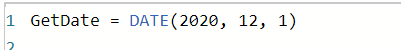It returns a datetime value as#### If Year value is less than 1900

Lets see the output of following DAX ,for year value 13 which is less than 1900.

`GetDate_YearLessthan1900 = DATE(13, 12, 1)`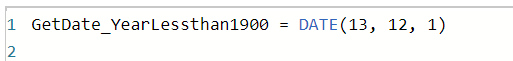It returns Datetime value that is 1/12/1913 12:00:00 AM , because value for year is 13 that is less than 1899 so it adds 1900 to the year so it becomes 1900+13 =1913.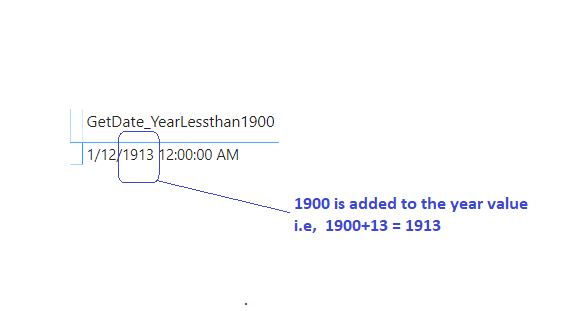#### IF year is greater than 9999 or less than 0

If a value for year is greater than 9999, lets say  when value of year is 10000  than Date function gives an error as shown.

`GetDate_YearGreaterThan9999 = DATE(10000, 2, 3)`IF a value for Year is less than 0 (negative), then Date function gives an following error.

`GetDate_NegativeYear = DATE(-1989, 2, 3)`#### IF a  value for Month is greater than 12

Lets see the following  DAX, when the month value greater than 12  are passed to date function.
`GetDate_DayGreaterthan12 = DATE(2020, 13, 1)`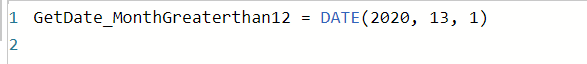As you can see, for month value which is greater than 12, it returns a datetime value that is 1st January 2021 because 13 months are added to the beginning of 2020.#### If a value for Month is Negative

Lets see the following DAX, when the negative month that is -10 are passed to date function.
`GetDate_NegativeMonth = DATE(2020, -10, 3)`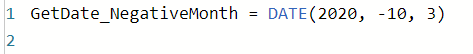As you can see, It retuns 3/2/2019 12:00:00 AM becuase 10 months are substracted from the begining of 2020.#### A value  for a day is greater than the last day of given month

Lets pass the value of day 33 for month 12,  It is greater than the last day of month 12 that is 31.
`GetDate_DayValueIsGreaterThanLastDayOfMonth = DATE(2020, 12, 33)`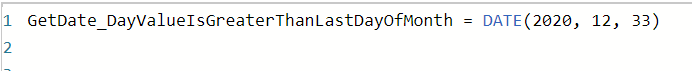As you can see,  It returns a datetime value  2/1/2019 12:00:00 AM because 33 days are added to the beginning of December 2020.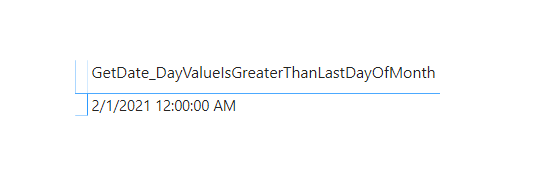#### A value  for a day is Negative

Lets pass the negative value  -3 for day.As you can see , It returns a datetime value = ’27/11/2020 12:00:00 AM’, because 3 days are subtracted from the beginning of December 2020.431 total views,  1 views today

This site uses Akismet to reduce spam. Learn how your comment data is processed.